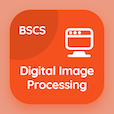Computer Science Courses Online

Digital Logic Design Practice Tests

Digital Logic Design Tests

The Book Analysis of Asynchronous Sequential Logic Multiple Choice Questions (MCQ Quiz) PDF, Analysis of Asynchronous Sequential Logic MCQs download to study online digital logic design certification courses. Study Asynchronous Sequential Logic Multiple Choice Questions and Answers (MCQs), Analysis of Asynchronous Sequential Logic quiz answers PDF for online computer science and engineering. The eBook Analysis of Asynchronous Sequential Logic MCQ App Download: design procedure of asynchronous sequential logic, analysis of asynchronous sequential logic, introduction to asynchronous sequential logic test prep for online software development courses.

The MCQ: Asynchronous circuits are useful in application where the input signals may PDF, Analysis of Asynchronous Sequential Logic App Download (Free) with change at any time, never change , and continuously change choices for online computer science and engineering. Practice analysis of asynchronous sequential logic quiz questions, download Amazon eBook (Free Sample) to learn free online courses.

## Logic Design: Analysis of Asynchronous Sequential Logic MCQs

MCQ: Asynchronous circuits are useful in application where the input signals may

A) change at any time
B) never change
C) both a and b
D) continuously change

MCQ: The table that is not a part of the asynchronous analysis procedure is

A) transition table
B) state table
C) flow table
D) excitation table

MCQ: What is the significant capacity/s of memory elements utilized in the sequential circuits?

A) storage of binary information
B) specify the state of sequential
C) both a and b
D) state machine

MCQ: In synchronous circuits, the present state is determined by

A) unclocked flip-flops
B) clocked flip-flops
C) flip-flops
D) latches

MCQ: The race in which stable state depends on an order is called

A) critical race
B) identical race
C) non critical race
D) defined race

### Practice Tests: Digital Logic Design Exam Prep

Download Digital Logic Design Quiz App, Digital Image Processing MCQ App, and DBMS MCQs App to install for Android & iOS devices. These Apps include complete analytics of real time attempts with interactive assessments. Download Play Store & App Store Apps & Enjoy 100% functionality with subscriptions!